#A dataset for question answering and reading comprehension from a set of Wikipedia articles

The Stanford Question Answering Dataset (SQuAD) consists of questions posed by crowd workers on a set of Wikipedia articles where the answer to every question is a segment of text, or span, from the corresponding reading passage. Unanswerable questions were added to the dataset for v2.0.

The "ContentElements" field contains eight options: "Dataset", "TrainingData", "ValidationData", "TrainingMetadata", "ValidationMetadata", "Data", "ColumnNames" and "ColumnDescriptions". "Dataset" contains the full dataset. Please note that data marked "Validation" in the ValidationRole field can have multiple possible answers for each question. "TrainingData" and "ValidationData" are formatted for standard question answering usage; for every question, only the first answer of the full dataset is selected. "TrainingMetadata" and "ValidationMetadata" contain the title of the Wikipedia article to which each question ID corresponds. "Data" contains the full dataset structured as an association. "ColumnNames" and "ColumnDescriptions" provide more information about the columns of the dataset.

Modifications from the original dataset: Data marked "Training" in the ValidationRole field corresponds to the Training Set v2.0 subset of the original dataset. Data marked "Validation" in the ValidationRole field corresponds to the Dev Set v2.0 subset of the original dataset. The original dataset is 0-indexed; in order be accurate in the Wolfram Language, 1 was added to the value of "AnswerPosition", as the Wolfram Language is 1-indexed.

## Examples

### Basic Examples

Retrieve the resource:

 In:=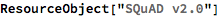Out=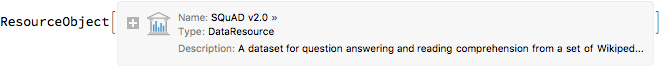Retrieve a sample of the dataset:

 In:=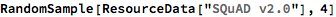Out=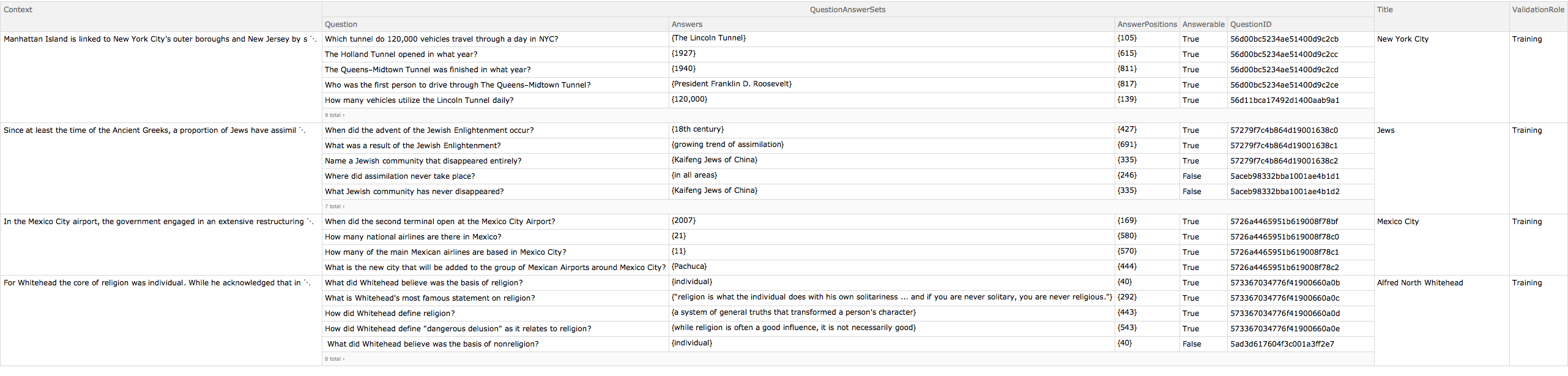### Working with Training and Validation Data

Obtain the first example from the training dataset:

 In:=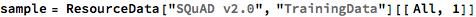Out=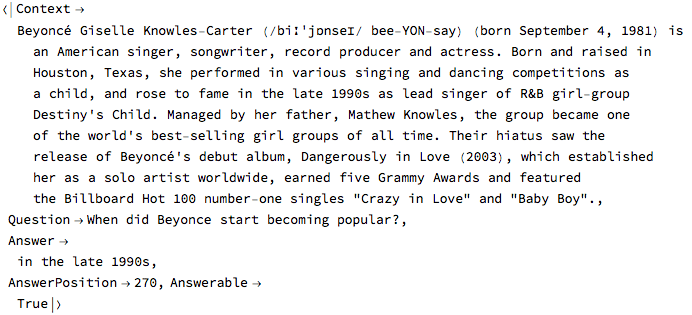View the question and answer associated with the passage:

 In:=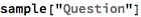Out=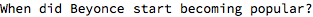In:=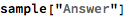Out=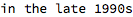"AnswerPosition" shows where the first character of the answer is located in the passage. The position of the last character can be computed:

 In:=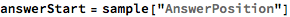Out=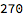In:=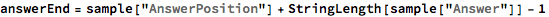Out=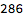Test that the span between the starting position and the ending position is equivalent to the answer:

 In:=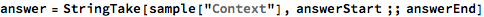Out=In:=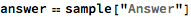Out=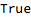In:=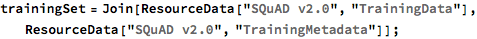There is a one-to-one correspondence between the data and the metadata; therefore, all lists will have the same length:

 In:=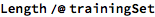Out=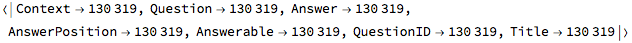View an example of the joined data:

 In:=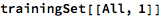Out=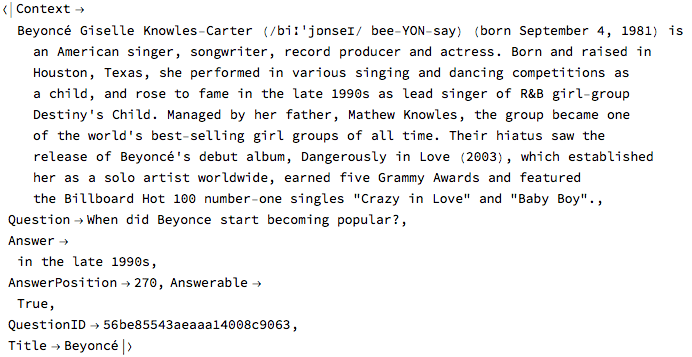### Aggregation

Set the types of questions:

 In:=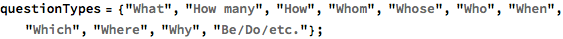Define the patterns corresponding to these questions:

 In:=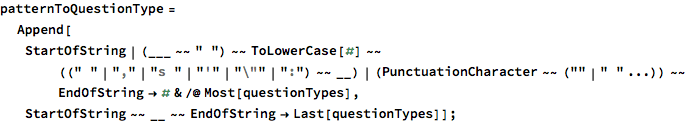Classify the questions from the SQuAD dataset:

 In:=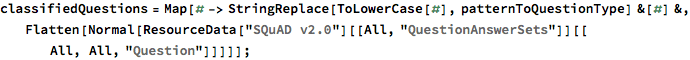Print the statistics about the question types:

 In:=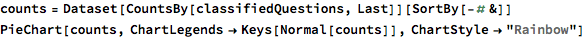Out=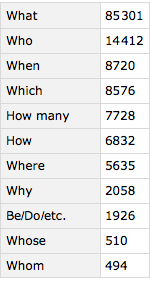Out=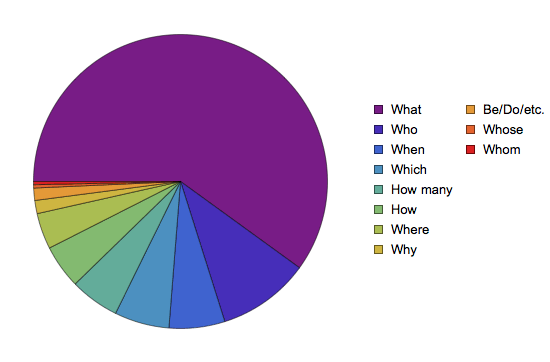Display some examples for each type of question:

 In:=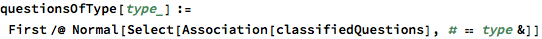In:=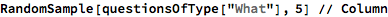Out=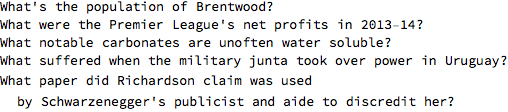In:=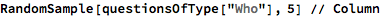Out=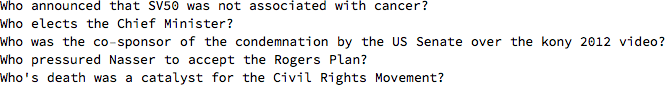In:=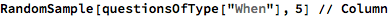Out=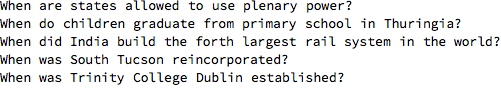In:=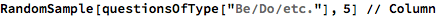Out=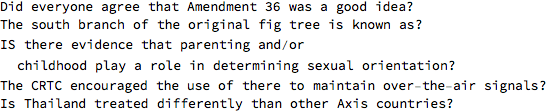### Dataset Size

Display the number of Wikipedia pages in the training and validation datasets:

 In:=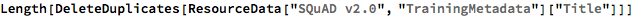Out=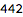In:=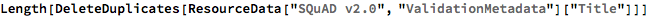Out=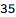Display the number of Wikipedia paragraphs in the training and validation datasets:

 In:=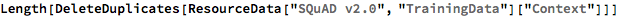Out=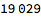In:=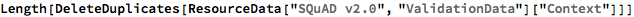Out=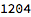Display the number of question/answer pairs in the training and validation datasets:

 In:=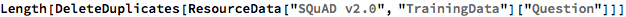Out=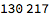In:=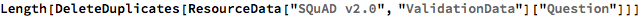Out=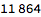Wolfram Research, "SQuAD v2.0" from the Wolfram Data Repository (2019) https://doi.org/10.24097/wolfram.32475.data